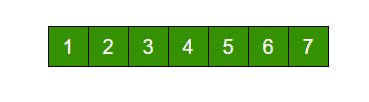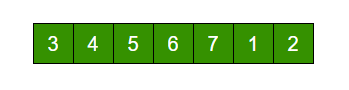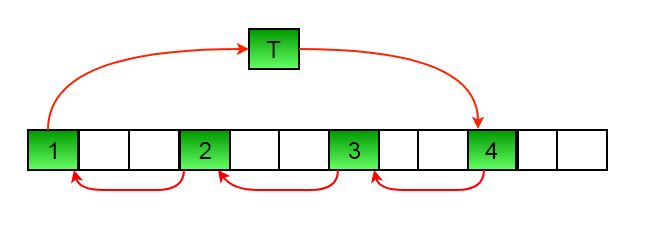GFG App
Open AppBrowser
Continue

# Java Program For Array Rotation

Write a function rotate(ar[], d, n) that rotates arr[] of size n by d elements.Rotation of the above array by 2 will make arrayMETHOD 1 (Using temp array)

```Input arr[] = [1, 2, 3, 4, 5, 6, 7], d = 2, n =7
1) Store d elements in a temp array
temp[] = [1, 2]
2) Shift rest of the arr[]
arr[] = [3, 4, 5, 6, 7, 6, 7]
3) Store back the d elements
arr[] = [3, 4, 5, 6, 7, 1, 2]```

Time complexity : O(n)
Auxiliary Space : O(d)

METHOD 2 (Rotate one by one)

```leftRotate(arr[], d, n)
start
For i = 0 to i < d
Left rotate all elements of arr[] by one
end```

To rotate by one, store arr in a temporary variable temp, move arr to arr, arr to arr …and finally temp to arr[n-1]

Let us take the same example arr[] = [1, 2, 3, 4, 5, 6, 7], d = 2
Rotate arr[] by one 2 times
We get [2, 3, 4, 5, 6, 7, 1] after first rotation and [ 3, 4, 5, 6, 7, 1, 2] after second rotation.

## Java

 `class` `RotateArray  ` `{ ` `    ``/*Function to left rotate arr[] of size n by d*/` `    ``void` `leftRotate(``int` `arr[], ``int` `d, ``int` `n)  ` `    ``{ ` `        ``int` `i; ` `        ``for` `(i = ``0``; i < d; i++) ` `            ``leftRotatebyOne(arr, n); ` `    ``} ` ` `  `    ``void` `leftRotatebyOne(``int` `arr[], ``int` `n)  ` `    ``{ ` `        ``int` `i, temp; ` `        ``temp = arr[``0``]; ` `        ``for` `(i = ``0``; i < n - ``1``; i++) ` `            ``arr[i] = arr[i + ``1``]; ` `        ``arr[i] = temp; ` `    ``} ` ` `  `    ``/* utility function to print an array */` `    ``void` `printArray(``int` `arr[], ``int` `size)  ` `    ``{ ` `        ``int` `i; ` `        ``for` `(i = ``0``; i < size; i++) ` `            ``System.out.print(arr[i] + ``" "``); ` `    ``} ` ` `  `    ``// Driver program to test above functions ` `    ``public` `static` `void` `main(String[] args)  ` `    ``{ ` `        ``RotateArray rotate = ``new` `RotateArray(); ` `        ``int` `arr[] = {``1``, ``2``, ``3``, ``4``, ``5``, ``6``, ``7``}; ` `        ``rotate.leftRotate(arr, ``2``, ``7``); ` `        ``rotate.printArray(arr, ``7``); ` `    ``} ` `} ` ` `  `// This code has been contributed by Mayank Jaiswal `

Output :

```3 4 5 6 7 1 2
```

Time complexity : O(n * d)
Auxiliary Space : O(1)
METHOD 3 (A Juggling Algorithm)
This is an extension of method 2. Instead of moving one by one, divide the array in different sets
where number of sets is equal to GCD of n and d and move the elements within sets.
If GCD is 1 as is for the above example array (n = 7 and d =2), then elements will be moved within one set only, we just start with temp = arr and keep moving arr[I+d] to arr[I] and finally store temp at the right place.

Here is an example for n =12 and d = 3. GCD is 3 and

```Let arr[] be {1, 2, 3, 4, 5, 6, 7, 8, 9, 10, 11, 12}

a)    Elements are first moved in first set – (See below diagram for this movement)arr[] after this step --> {4 2 3 7 5 6 10 8 9 1 11 12}

b)    Then in second set.
arr[] after this step --> {4 5 3 7 8 6 10 11 9 1 2 12}

c)    Finally in third set.
arr[] after this step --> {4 5 6 7 8 9 10 11 12 1 2 3}
```

## Java

 `class` `RotateArray  ` `{ ` `    ``/*Function to left rotate arr[] of size n by d*/` `    ``void` `leftRotate(``int` `arr[], ``int` `d, ``int` `n)  ` `    ``{ ` `        ``int` `i, j, k, temp; ` `        ``for` `(i = ``0``; i < gcd(d, n); i++)  ` `        ``{ ` `            ``/* move i-th values of blocks */` `            ``temp = arr[i]; ` `            ``j = i; ` `            ``while` `(``true``)  ` `            ``{ ` `                ``k = j + d; ` `                ``if` `(k >= n)  ` `                    ``k = k - n; ` `                ``if` `(k == i)  ` `                    ``break``; ` `                ``arr[j] = arr[k]; ` `                ``j = k; ` `            ``} ` `            ``arr[j] = temp; ` `        ``} ` `    ``} ` ` `  `    ``/*UTILITY FUNCTIONS*/` `     `  `    ``/* function to print an array */` `    ``void` `printArray(``int` `arr[], ``int` `size)  ` `    ``{ ` `        ``int` `i; ` `        ``for` `(i = ``0``; i < size; i++) ` `            ``System.out.print(arr[i] + ``" "``); ` `    ``} ` ` `  `    ``/*Function to get gcd of a and b*/` `    ``int` `gcd(``int` `a, ``int` `b)  ` `    ``{ ` `        ``if` `(b == ``0``) ` `            ``return` `a; ` `        ``else` `            ``return` `gcd(b, a % b); ` `    ``} ` ` `  `    ``// Driver program to test above functions ` `    ``public` `static` `void` `main(String[] args) { ` `        ``RotateArray rotate = ``new` `RotateArray(); ` `        ``int` `arr[] = {``1``, ``2``, ``3``, ``4``, ``5``, ``6``, ``7``}; ` `        ``rotate.leftRotate(arr, ``2``, ``7``); ` `        ``rotate.printArray(arr, ``7``); ` `    ``} ` `} ` ` `  `// This code has been contributed by Mayank Jaiswal `

Output :

```3 4 5 6 7 1 2
```

Time complexity : O(n)
Auxiliary Space : O(1)

Please refer complete article on Program for array rotation for more details!

My Personal Notes arrow_drop_up